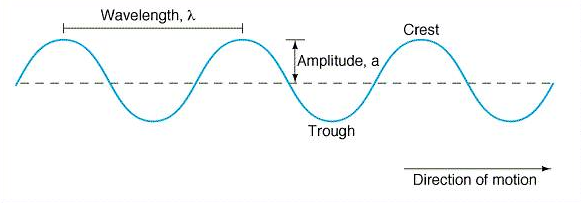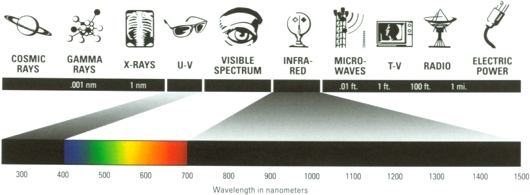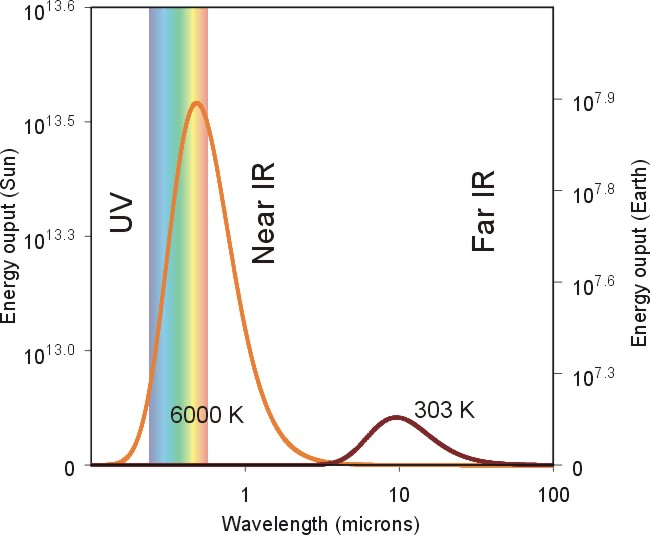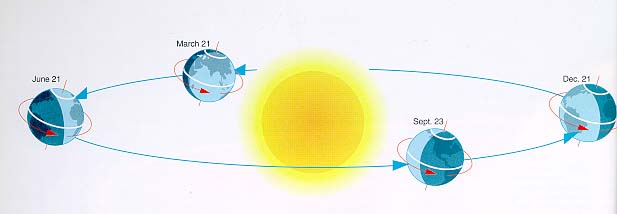>> Lecture Two: Radiation << This lecture covers some of the basics of electromagnetic radiation and the electromagnetic spectrum in relation to meteorology.

>> Lecture Two Outline <<

>> Described by "wavelength" and "amplitude"Figure 1: Above is an illustration of the basic structure of a wave.

All waves can be described by the same two parameters, the wavelength and amplitude.  The wavelength is defined as the distance between one peak or crest of a wave and the next corresponding peak or crest.  The vertical distance from the midpoint of a wave to a crest or trough is called the amplitude.  Figure 1, seen above, details the basic structure of a wave.Figure 2: The image above details the array of wavelengths that make up the electromagnetic spectrum.

The electromagnetic spectrum, seen above in Figure 2, is a collection of wavelengths of radiation ranging from radio waves to cosmic rays.

>> Wavelength of peak emissionFigure 3: This figure shows the wavelength of peak emission for the sun and the earth.  Notice that the peak wavelength for the sun is at a shorter wavelength due to its higher temperature.

While all objects emit radiation at all wavelengths, the amount of radiation emitted usually peaks at a certain wavelength.  The wavelength of peak emission depends on the temperature of the object emitting radiation.  A higher temperature will cause the wavelength of peak emission to be at a shorter wavelength.

Temperature

>> A measure of the average kinetic energy of the molecules of an object

 Please download Macromedia Shockwave in order to view this demonstration. Figure 4: The application above shows the relationship between the temperature of an object and the average kinetic energy of its molecules.  If you do not have the Macromedia Shockwave player on your computer, click here to download it.

The temperature of an object is directly related to the average speed of the molecules that make up the object.  If the temperature of an object is high, its molecules will move at a greater speed and will, therefore, have a greater average kinetic energy.  This can be seen in the demonstration to the left.

>> The greater the average kinetic energy, the higher the temperature
>> As temperature increases, the amount of emitted energy (radiation) increases, while the wavelength of peak emission decreases.

The increase in emitted energy and decrease in the wavelength of peak emission can be seen by revisiting Figure 3.  In this figure, the amount of radiation emitted is plotted as a function of the wavelength of radiation for both the sun and the earth.  If you compare the amount of radiation emitted by the two celestial bodies, you can clearly see that the sun emits much more radiation than the earth.  You also notice that the wavelength of peak emission for the sun is significantly less than that of the earth.  Both of these effects are a result of the sun having a much higher temperature than the earth.

>> Absorptivity (color)
>> Intensity (sun angle)
>> Net heating and cooling

Absorptivity: Transparent versus Opaque

>> Greenhouse gases (CO2 and H2O)

Annual Cycle: Tilt of the Earth's Axis

>> Tilt and Seasons

The four season experienced by many people around the world are due solely to the tilt of the earth's axis.  As the earth revolves around the sun, the tilt of the earth's axis causes the planet to be oriented differently with respect to the sun.  This can be seen in Figure 5 below.Figure 5: The image above shows how the tilt of the earth's axis causes the four different seasons across the planet.

As we all know, with changing seasons comes changing weather and temperatures.  The changing weather and temperatures are also a direct result of the tilt of the earth's axis.  The seasonal change in temperatures can be seen in Figure 6 below.

 Please download the Quicktime plug-in to view this example. Figure 6: The figure above shows the progression of global temperatures throughout the year.  The changes are based on the tilt of the earth's axis and the resulting seasons.

Home

Quick Notes

Copyright 2004 and later by Thomas P. Harrington